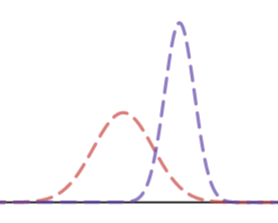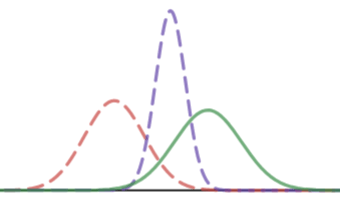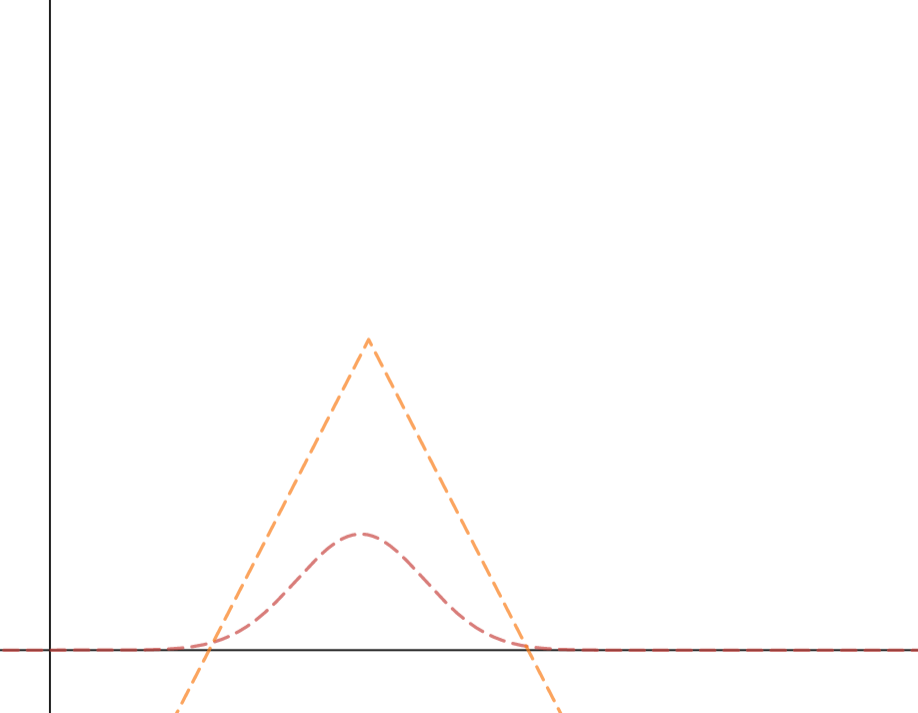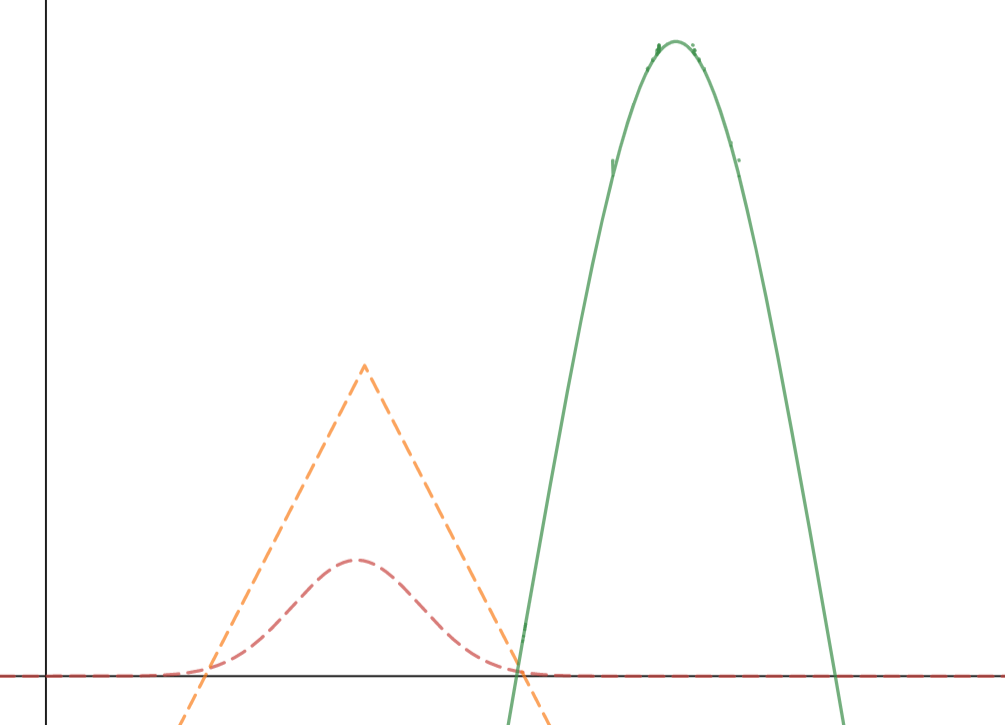# Another way to smoosh two Bell Curves together

Previously, we looked at one way of combining two Bell Curve (i.e. Gaussian distributions) together to make a third — multiplication.

There are other ways to do this, though. The best known (and as far as I can tell, the most important) is convolution. So, here are two Gaussian distributions, and what you get when you convolute(?) them:Formally, this process takes two functions —$f(x), g(x)$ — and then produces a new distribution defined in the following way:$(f * g)(t) = \int_{-\infty}^{+\infty}f(x)g(t-x)dx$

I have been struggling with this idea for several months, but just a few days ago I made some progress.

Convolution is often described as a blurring process — it blurs one distribution according to another one. That’s how Terry Tao describes it in this Math Overflow post:

If one thinks of functions as fuzzy versions of points, then convolution is the fuzzy version of addition (or sometimes multiplication, depending on the context). The probabilistic interpretation is one example of this (where the fuzz is a a probability distribution), but one can also have signed, complex-valued, or vector-valued fuzz, of course.

I have a hard time seeing the “blurring” in the images above. To really see it, I have to change the initial functions. For example, consider the convolution of a Gaussian and linear function (with restricted domain). Before convolution……and after.Just playing around with the calculator a bit more, here is another before/after pair.I first learned about convolution a few months ago, and it was explained to me in terms of blurring. The thing about this “intuition building” metaphor is that I’ve been sitting with it since then, and it hasn’t helped me feel comfortable with it at all. It was only last week when I came across the far more prosaic meaning of convolution that things started to click for me. Because besides for whatever blurring convolution represents, it also represents the sum of two independent random things.

(What follows is lifted from this excellent text.)

Suppose you have two dice, both six-sided, both fair. There is an equal chance of rolling 1, 2, 3, 4, 5 or 6 with each die — a uniform probability distribution.$P(x) = \frac{1}{6}$ whether$x = 1, 2, 3, 4, 5, 6$.

What would the distribution of the sums of the rolls look like?

The calculations start relatively simply, but check out the structure. For example, this is the calculation we have to do to find the chances of rolling a a 3:$P(1)P(2) + P(2)P(1)$

The chances of rolling a 4:$P(1)P(3) + P(2)P(2) + P(3)P(1)$

The chances of rolling a 5:$P(1)P(5-1) + P(2)P(5-2) + P(3)P(5-3) + P(4)P(5-4)$

The chances of rolling n:$P(1)P(n - 1) + P(2)P(n - 2) + ... + P(n-1)P(n - (n- 1))$

So! Using the language of summation, we can summarize this process as so:$P(sum = n) = \sum P(k) \cdot P(n - k)$

We might as well take the last step of calling this process “convolution,” because it’s just the discrete version of the integral from above!:$(f * g)(t) = \int_{-\infty}^{+\infty}f(x)g(t-x)dx$

***

Mathematically, there is a lot of interesting stuff to continue exploring with convolutions. Not all convolutions are defined, there’s a connection to Fourier transforms (another thing I don’t understand yet), there are discrete problems to solve in the text (what about different dice?), and so on and so on.

Briefly, though: why didn’t the blurring metaphor help me? I don’t think it’s such a mystery. It’s because while blurring is easy to understand, that image was totally disconnected from the underlying calculation. Why should that complicated integral be related to the process of blurring?

Now, I have a better understanding. (Blurring X and Y sort of is like finding the probability of X + Y.)

In school math, a lot of teachers bemoan their students’ lack of conceptual understanding, and it’s generally felt that procedural understanding is more obtainable. In my life as a learner of mathematics I usually feel it’s the other way around. When I read articles in Quanta Magazine or books about mathematics I haven’t yet studied I can usually follow the exposition but am left feeling a bit empty. Yes, I can follow the metaphors (“Imagine a number as a little bird; those birds fly together in flocks; but what happens when a bird has children? do they rejoin the flock? where? etc.”) but what have I learned?

Popular exposition of mathematics is maybe more difficult than exposition of other subjects simply because of the necessity of some kind of metaphor that brings the abstract to life. What results is a kind of understanding, but something far from the whole thing, and the best mathematical exposition also leaves me feeling jealous of those who can reach past the metaphors and grasp the thing itself.

That’s not to say that math exposition for popular audiences isn’t valuable — it is! Most people aren’t ever going to reach that deeper, unified understanding. I certainly won’t, most of the time! But for convolution, I feel a step closer.

## One thought on “Another way to smoosh two Bell Curves together”

1. I heart this.

More precisely, I heart investigation of math that also has an eye toward what the experience of learning math says about teaching math. (Especially from an experienced educator who is tuned into wider conversations about teaching math and can put their own experience of learning math in dialogue with those conversations.)

Convolve, FWIW.